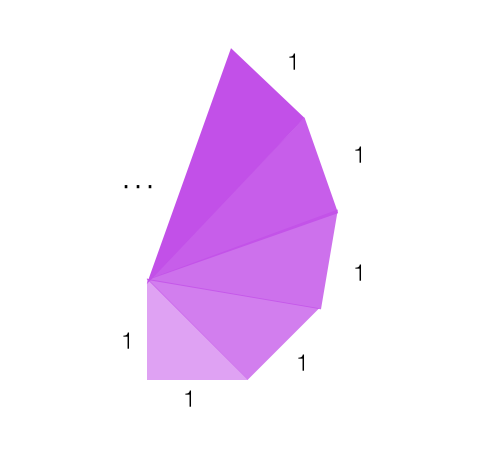## More on Iterated Functions

2017 April 4

After using iterated functions to calculate the amortization formulas, they've caught my interest again (no pun intended). This post, unfortunately, will be little more than a list of questions and concerns about iterated functions that I have been posing to myself, aside from one enormously helpful realization.

The thing that I noticed was that there is a whole class of functions that can be easily iterated: functions of the form

where $g(x)$ and $h(x)$ are other functions. Something very special happens when we compose it with itself:

The $g$ and $g^{-1}$ undo each other and we are left with

In fact, this beautiful property holds for any number of iterations since $g$ and $g^{-1}$ undo each other every time $f(x) is iterated: This is incredibly useful, since it allows us to iterate any function of this form, so long as we know how to iterate$h(x)$. One classic example of the iteration of a function of this type is the right triangle spiral problem. In this problem, one starts off with an isoscles right triangle with unit leg length. Then one constructs another right triangle with one leg of unit length and one leg made of the hypotenuse of the previous triangle. This process is repeated to create more and more triangles, and the problem is to find the length of the longest leg of the nth triangle.We can see that$f(1)=1$easily, and that, by the pythagorean theorem, the leg length of each triangle is the hypotenuse length of the previous triangle. This means that the function mapping each leg length to the leg length of the next triangle is and the leg length of the nth triangle will be We can rearrange$f(x)$so that it is in the form where$g(x)=\sqrt{x}$and$h(x)=x+1$, so when we iterate$f(x)$, we will get because$h^n(x)=x+n$. Now we can find$f^{n-1}(1)$: and the long leg length of the nth triangle is given by$\sqrt n$. Another example is the iteration of the "softplus" function which turns out to be since$\ln$and$e^x$cancel each other, but the 1s stack up with each iteration. That's the development that I've made. Here are my questions that have yet to be answered: 1. It seems that many polynomial functions simply won't iterate nicely, such as$f(x)=x^2+1$. How can I determine whether or not a polynomial has an iteration formula, and what are the iteration formulas for the polynomials for which I can do this? If I can't find iteration formulas for some polynomial functions, can I find fractional iterates? 2. Rational functions of the form$f(x)=\frac{ax+b}{cx+d}$iterate in a really interesting way that seems to be connected somehow to the fibonacci numbers. Can I use this to write an iteration formula for functions of that form? 3. Some functions, like the function$f(x)=-x$, seem to not have any half-iterates that don't involve imaginary numbers. How do I determine the existence of a function's half-iterate? 4. One interesting class of functions is the class of involutory functions, or functions that are their own inverses. How many of these functions are there? What are their properties? Are their functions with the property that$f^3(x)=x$or$f^4(x)=x\$?

5. Some functions, when iterated, aren't perfectly represented by their iteration formula because of discrepancies in domain and range. How can I detect such discrepancies? Can I formulate a general rule?

6. Are there ways that I can find a large iterate of a function for a specific value without writing it out by hand or finding an iteration formula?

I hope to revisit these topics in later posts.